# GMAT Math : Properties of Integers

## Example Questions

← Previous 1 3 4 5 6 7 8

### Example Question #1 : Understanding The Properties Of Integers

What is the sum of the prime numbers that are greater than 50 but less than 60?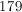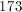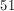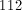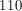Explanation:

A prime number is only divisible by the number 1 and itself. Of the integers between 50 and 60, all of the even integers are also divisible by the number 2 so they are not prime numbers. The integers 51 and 57 are divisible by 3. The integer 55 is divisible by 5. The only integers that are prime are 53 and 59. The sum of these two integers is 112.

### Example Question #161 : Arithmetic

What is the product of the four smallest prime numbers?

945

0

30

210

1155

210

Explanation:

We must remember that 0 and 1 are NOT prime numbers, but 2 is.

The four smallest prime numbers are 2, 3, 5, and 7. Then 2 * 3 * 5 * 7 = 210.

Note: There are NO negative prime numbers, so we don't have to look for tiny, negative numbers here.

### Example Question #1 : Integers And Types Of Numbers

If a and b are even integers, what must be odd?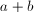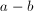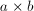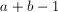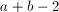Explanation:

The sum (or difference) or 2 even integers is even. Similarly, the product (or quotient) of 2 even integers is also even; therefore the answer must be, which can be easily checked by plugging in any two even numbers.

For example, if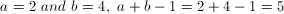, which is odd.

### Example Question #1 : Understanding The Properties Of Integers

Three consecutive numbers add up to 36.  What is the smallest number?Explanation:

The sum of 3 consecutive numbers would be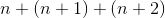which simplifies into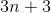Set that equation equal to 36 and solve.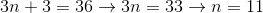### Example Question #4 : Properties Of Integers

Becky has to choose from 4 pairs of pants, 6 shirts, and 2 pairs of shoes for an interview. If an outfit consists of 1 pair of pants, 1 pair of shoes, and 1 shirt, how many options does she have?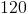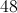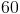Explanation:

To find total number, multiply the number of each item.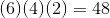### Example Question #1 : Properties Of Integers

What is the greatest prime factor of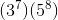Explanation:

The only prime factors are 3 and 5, therefore, 5 will be the greatest prime factor.

### Example Question #1 : Properties Of Integers

Solve: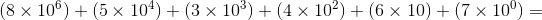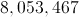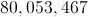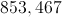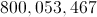Explanation: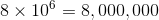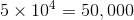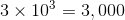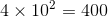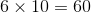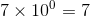The sum is 8,053,467

### Example Question #1 : Understanding The Properties Of Integers

If a positive integeris divided by another positive integer, then the quotient is 6, and there is no remainder.

Which of these choices is a possible value of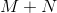?

Each of the other choices is a possible value of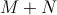.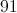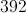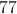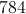Each of the other choices is a possible value of.

Explanation:

The conditions of the problem can be rewritten as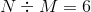, or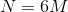.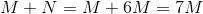, meaning that the sum of the two numbers is a multiple of 7. Each of the given choices is a multiple of 7, so any of them can be.

### Example Question #171 : Arithmetic

What is the first digit in the base-six representation of the number 936?Explanation: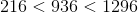, or, equivalently,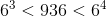. This makes 936 a four-digit number when written in base six. The first digit is equal to the number of times 216 divides into 936.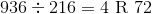,

4 is the first digit of this number.

### Example Question #8 : Understanding The Properties Of Integers

What is the last digit in the base-eight representation of the number 735?Explanation:

Divide 735 by 8. The remainder is the last digit of the base-eight representation.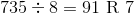The correct choice is 7.

← Previous 1 3 4 5 6 7 8

### All GMAT Math Resources